If there is one prayer that you should pray/sing every day and every hour, it is the LORD's prayer (Our FATHER in Heaven prayer)
It is the most powerful prayer. A pure heart, a clean mind, and a clear conscience is necessary for it.
- Samuel Dominic Chukwuemeka

For in GOD we live, and move, and have our being. - Acts 17:28

The Joy of a Teacher is the Success of his Students. - Samuel Chukwuemeka

# Solved Examples on Right TrianglesVerify your answers with these Calculators as applicable.

For ACT Students
The ACT is a timed exam...60 questions for 60 minutes
This implies that you have to solve each question in one minute.
Some questions will typically take less than a minute a solve.
Some questions will typically take more than a minute to solve.
The goal is to maximize your time. You use the time saved on those questions you solved in less than a minute, to solve the questions that will take more than a minute.
So, you should try to solve each question correctly and timely.
So, it is not just solving a question correctly, but solving it correctly on time.
Please ensure you attempt all ACT questions.
There is no negative penalty for a wrong answer.

For SAT Students
Any question labeled SAT-C is a question that allows a calculator.
Any question labeled SAT-NC is a question that does not allow a calculator.

For JAMB Students
Calculators are not allowed. So, the questions are solved in a way that does not require a calculator.

For WASSCE Students
Any question labeled WASCCE is a question for the WASCCE General Mathematics
Any question labeled WASSCE:FM is a question for the WASSCE Further Mathematics/Elective Mathematics

For GCSE and Malta Students
All work is shown to satisfy (and actually exceed) the minimum for awarding method marks.
Calculators are allowed for some questions. Calculators are not allowed for some questions.

For NSC Students
For the Questions:
Any space included in a number indicates a comma used to separate digits...separating multiples of three digits from behind.
Any comma included in a number indicates a decimal point.
For the Solutions:
Decimals are used appropriately rather than commas
Commas are used to separate digits appropriately.

Solve all questions.
State the reasons for every step.
Show all work.

(1.) ACT Which of the following is the sine of the smallest angle in a right triangle with side lengths $7$, $24$, and $25$ inches, respectively?

$hyp = 25 \\[3ex] short = 7 \\[3ex] middle = 24 \\[3ex] Let\:\: \alpha \lt \beta \lt 90 \\[3ex]$ side length is proportional to angle measure...Triangle Theorem $10$

$7 \:\:faces\:\: \alpha = short \\[3ex] 24 \:\:faces\:\: \beta = middle \\[3ex] 25 \:\:faces\:\: 90 = hyp \\[3ex] SOHCAHTOA \\[3ex] \sin \alpha = \dfrac{opp}{hyp} \\[5ex] \sin \alpha = \dfrac{7}{25} \\[5ex] \alpha = \sin^{-1} \left(\dfrac{7}{25}\right) \\[5ex] \sin \beta = \dfrac{24}{25} \\[5ex] \beta = \sin^{-1} \left(\dfrac{24}{25}\right) \\[5ex] \dfrac{24}{25} \gt \dfrac{7}{25} \\[5ex] smallest\:\: angle = \alpha \\[3ex] \therefore \sin \alpha = \dfrac{7}{25}$
(2.) ACT What is the length, in centimeters (cm), of the hypotenuse of a right triangle with legs measuring $5$ cm and $12$ cm?

$hyp^2 = short^2 + middle^2 ...Pythagorean\:\: Theorem \\[3ex] short = 5 \\[3ex] middle = 12 \\[3ex] hyp^2 = 5^2 + 12^2 \\[3ex] hyp^2 = 25 + 144 = 169 \\[3ex] hyp = \sqrt{169} \\[3ex] hyp = 13cm$
(3.) ACT The ratio of the lengths of the sides of a right triangle is $1:\sqrt{3}:2$.
What is the sine of the triangle's smallest angle?

$1:\sqrt{3}:2 \\[3ex] short = 1 \\[3ex] middle = \sqrt{3} \\[3ex] hyp = 2 \\[3ex] Let\:\: \alpha \lt \beta \lt 90 \\[3ex]$ side length is proportional to angle measure...Triangle Theorem $10$

$1 \:\:faces\:\: \alpha = opp \\[3ex] 2 \:\:faces\:\: 90 = hyp \\[3ex] SOHCAHTOA \\[3ex] \sin \alpha = \dfrac{opp}{hyp} \\[5ex] \sin \alpha = \dfrac{1}{2}$
(4.) ACT The lengths of the legs of a right triangle are $4$ miles and $5$ miles, respectively.
Which of the following lengths, in miles, is closest to that of the hypotenuse of the right triangle?

$hyp^2 = leg^2 + leg^2 ...Pythagorean\:\: Theorem \\[3ex] leg = short = 4 \\[3ex] leg = middle = 5 \\[3ex] hyp^2 = 4^2 + 5^2 \\[3ex] hyp^2 = 16 + 25 = 41 \\[3ex] hyp = \sqrt{41} \\[3ex] hyp \approx 6.4 \:\:miles$
(5.) ACT For an angle with measure $\alpha$ in a right triangle,

$\sin \alpha = \dfrac{180}{181}$ and $\tan \alpha = \dfrac{180}{19}$

What is the value of $\cos \alpha$?

$\tan \alpha = \dfrac{\sin \alpha}{\cos \alpha} ...Quotient\:\: Identity \\[5ex] \implies \cos \alpha = \dfrac{\sin \alpha}{\tan \alpha} \\[5ex] \sin \alpha = \dfrac{180}{181} \\[5ex] \tan \alpha = \dfrac{180}{19} \\[5ex] \cos \alpha = \dfrac{180}{181} \div \dfrac{180}{19} \\[5ex] \cos \alpha = \dfrac{180}{181} * \dfrac{19}{180} \\[5ex] \cos \alpha = \dfrac{19}{181}$
(6.) JAMB A straight line makes an angle of $30^\circ$ with the positive $x-axis$ and cuts the $y-axis$ at $y = 5$.
Find the equation of the straight line.

$A.\:\: y = \dfrac{1}{10}x + 5 \\[5ex] B.\:\: y = x + 5 \\[3ex] C.\:\: \sqrt{3}y = -x + 5\sqrt{3} \\[3ex] D.\:\: \sqrt{3}y = x + 5\sqrt{3} \\[3ex]$

JAMB is a timed exam that does not allow the use of calculators.
It is important you memorize the trigonometric functions of special angles.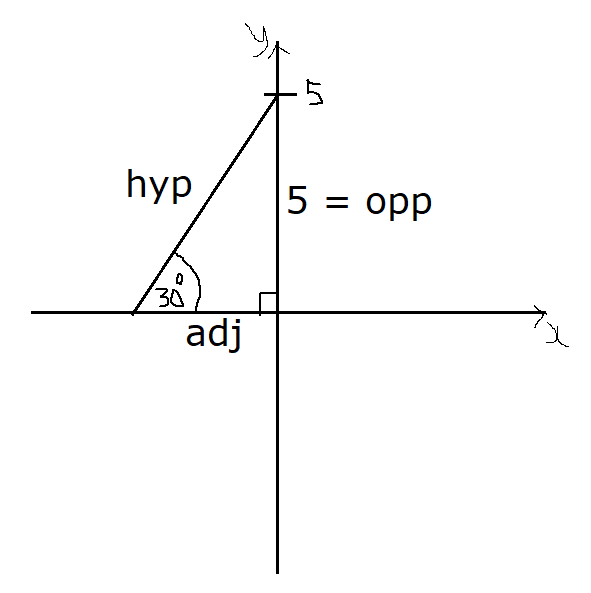$SOHCAHTOA \\[3ex] opp = 5 \\[3ex] \tan 30 = \dfrac{1}{\sqrt{3}} = \dfrac{opp}{adj} ...Unit\:\: Circle \\[5ex] \dfrac{1}{\sqrt{3}} = \dfrac{5}{adj} \\[5ex] 1 * adj = 5 * \sqrt{3} \\[3ex] adj = 5\sqrt{3} \\[3ex] m = \dfrac{\Delta y}{\Delta x} = \dfrac{5}{5\sqrt{3}} ...Slope\:\: Formula \\[5ex] m = \dfrac{1}{\sqrt{3}} \\[5ex] y = mx + b ...eqn. \:\:of\:\: a\:\: straight\:\: line \\[3ex] b = 5 \\[3ex] y = \dfrac{1}{\sqrt{3}}x + 5 \\[5ex] LCD = \sqrt{3} \\[3ex] \sqrt{3} * y = \sqrt{3} * \dfrac{1}{\sqrt{3}}x + \sqrt{3} * 5 \\[5ex] \sqrt{3}y = x + 5\sqrt{3}$
(7.) Given that $\tan \phi = 3$, find the other trigonometric functions.

$SOHCAHTOA \\[3ex] \tan \phi = \dfrac{opp}{adj} = 3 = \dfrac{3}{1} \\[5ex] opp = 3 \\[3ex] adj = 1 \\[3ex] \cot \phi = \dfrac{1}{\tan \phi} ...Reciprocal\:\: Identity \\[5ex] \cot \phi = \dfrac{1}{3} \\[5ex]$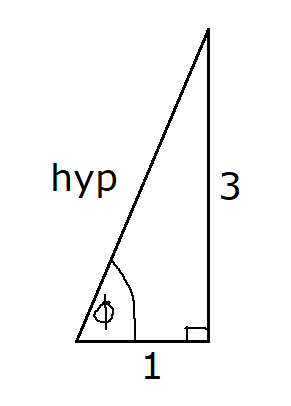$hyp^2 = adj^2 + opp^2 ...Pythagorean\:\: Theorem \\[3ex] hyp^2 = 1^2 + 3^2 \\[3ex] hyp^2 = 1 + 9 \\[3ex] hyp^2 = 10 \\[3ex] hyp = \sqrt{10} \\[3ex] \sin \phi = \dfrac{opp}{hyp} \\[5ex] \sin \phi = \dfrac{3}{\sqrt{10}} \\[5ex] \sin \phi = \dfrac{3}{\sqrt{10}} * \dfrac{\sqrt{10}}{\sqrt{10}} \\[5ex] \sin \phi = \dfrac{3\sqrt{10}}{10} \\[5ex] \csc \phi = \dfrac{1}{\sin \phi} ...Reciprocal\:\: Identity \\[5ex] \csc \phi = 1 \div \sin \phi \\[3ex] \csc \phi = 1 \div \dfrac{3}{\sqrt{10}} \\[5ex] \csc \phi = 1 * \dfrac{\sqrt{10}}{3} \\[5ex] \csc \phi = \dfrac{\sqrt{10}}{3} \\[5ex] \cos \phi = \dfrac{adj}{hyp} \\[5ex] \cos \phi = \dfrac{1}{\sqrt{10}} \\[5ex] \cos \phi = \dfrac{1}{\sqrt{10}} * \dfrac{\sqrt{10}}{\sqrt{10}} \\[5ex] \cos \phi = \dfrac{\sqrt{10}}{10} \\[5ex] \sec \phi = \dfrac{1}{\cos \phi} ...Reciprocal\:\: Identity \\[5ex] \sec \phi = 1 \div \cos \phi \\[3ex] \sec \phi = 1 \div \dfrac{\sqrt{10}}{10} \\[5ex] \sec \phi = 1 * \dfrac{10}{\sqrt{10}} \\[5ex] \sec \phi = \dfrac{10}{\sqrt{10}} * \dfrac{\sqrt{10}}{\sqrt{10}} \\[5ex] \sec \phi = \dfrac{10\sqrt{10}}{10} \\[5ex] \sec \phi = \sqrt{10}$
(8.) Given the function value of the acute angle, find the other five trigonometric function values.

$\cos \alpha = \dfrac{\sqrt{3}}{3}$

$SOHCAHTOA \\[3ex] \cos \alpha = \dfrac{\sqrt{3}}{3} = \dfrac{adj}{hyp} \\[5ex] adj = \sqrt{3} \\[3ex] hyp = 3 \\[3ex] \sec \alpha = \dfrac{1}{\cos \alpha} ...Reciprocal\:\: Identity \\[5ex] \sec \alpha = 1 \div \cos \alpha \\[3ex] \sec \alpha = 1 \div \dfrac{\sqrt{3}}{3} \\[5ex] \sec \alpha = 1 * \dfrac{3}{\sqrt{3}} \\[5ex] \sec \alpha = \dfrac{3}{\sqrt{3}} * \dfrac{\sqrt{3}}{\sqrt{3}} \\[5ex] \sec \alpha = \dfrac{3\sqrt{3}}{3} \\[5ex] \sec \alpha = \sqrt{3} \\[3ex]$$middle = opp \\[3ex] hyp^2 = adj^2 + opp^2 ...Pythagorean\:\: Theorem \\[3ex] opp^2 = hyp^2 - adj^2 \\[3ex] opp^2 = 3^2 - (\sqrt{3})^2 \\[3ex] opp^2 = 9 - 3 \\[3ex] opp^2 = 6 \\[3ex] opp = \sqrt{6} \\[3ex] \sin \alpha = \dfrac{opp}{hyp} \\[5ex] \sin \alpha = \dfrac{\sqrt{6}}{3} \\[5ex] \csc \alpha = \dfrac{1}{\sin \alpha} ...Reciprocal\:\: Identity \\[5ex] \csc \alpha = 1 \div \sin \alpha \\[3ex] \csc \alpha = 1 \div \dfrac{\sqrt{6}}{3} \\[5ex] \csc \alpha = 1 * \dfrac{3}{\sqrt{6}} \\[5ex] \csc \alpha = \dfrac{3}{\sqrt{6}} * \dfrac{\sqrt{6}}{\sqrt{6}} \\[5ex] \csc \alpha = \dfrac{3\sqrt{6}}{6} \\[5ex] \csc \alpha = \dfrac{\sqrt{6}}{2} \\[5ex] \tan \alpha = \dfrac{opp}{adj} \\[5ex] \tan \alpha = \dfrac{\sqrt{6}}{\sqrt{3}} \\[5ex] \tan \alpha = \dfrac{\sqrt{6}}{\sqrt{3}} * \dfrac{\sqrt{3}}{\sqrt{3}} \\[5ex] \tan \alpha = \dfrac{\sqrt{6} * \sqrt{3}}{\sqrt{3} * \sqrt{3}} \\[5ex] \tan \alpha = \dfrac{\sqrt{6 * 3}}{3} \\[5ex] \tan \alpha = \dfrac{\sqrt{18}}{3} \\[5ex] \tan \alpha = \dfrac{\sqrt{9} * \sqrt{2}}{3} \\[5ex] \tan \alpha = \dfrac{3\sqrt{2}}{3} \\[5ex] \tan \alpha = \sqrt{2} \\[3ex] \cot \alpha = \dfrac{1}{\tan \alpha} ...Reciprocal\:\: Identity \\[5ex] \cot \alpha = \dfrac{1}{\sqrt{2}} \\[5ex] \cot \alpha = \dfrac{1}{\sqrt{2}} * \dfrac{\sqrt{2}}{\sqrt{2}} \\[5ex] \cot \alpha = \dfrac{\sqrt{2}}{2}$
(9.) ACT If $\sin A = \dfrac{4}{5}$, then which of the following could be $\tan A$?

$F.\:\: \dfrac{1}{4} \\[5ex] G.\:\: \dfrac{3}{4} \\[5ex] H.\:\: 1 \\[3ex] J.\:\: \dfrac{4}{3} \\[5ex] K.\:\: 4$

$SOHCAHTOA \\[3ex] \sin A = \dfrac{4}{5} \\[5ex] opp = 4 \\[3ex] hyp = 5 \\[3ex] hyp^2 = adj^2 + opp^2 ...Pythagorean\:\: Theorem \\[3ex] adj^2 = hyp^2 - opp^2 \\[3ex] adj^2 = 5^2 - 4^2 \\[3ex] adj^2 = 25 - 16 \\[3ex] adj^2 = 9 \\[3ex] adj = \sqrt{9} = 3 \\[3ex] \tan A = \dfrac{opp}{adj} \\[5ex] \tan A = \dfrac{4}{3}$
(10.)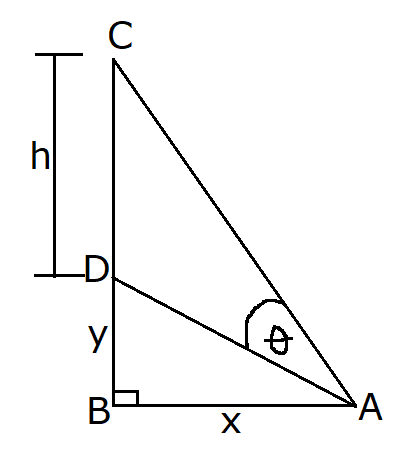Zephaniah's eye is at a point $A$, looking at a mural of height, with the bottom of the mural $y$ feet above the eye as seen in the figure.
The eye is $x$ feet from the wall.
(a.) Write an expression for $\theta$ in terms of $x, y$, and $h$.
(b.) Then, evaluate the expression when $x = 10ft$, $y = 5ft$, and $h = 16ft$.

There are two right triangles in this figure: $\triangle DBA$ and $\triangle CBA$

$(a.) \\[3ex] \underline{\triangle DBA} \\[3ex] \tan \angle DAB = \dfrac{y}{x} ... SOHCAHTOA \\[5ex] \angle DAB = \tan^{-1} \left(\dfrac{y}{x}\right) \\[5ex] \underline{\triangle CBA} \\[3ex] \tan (\theta + \angle DBA) = \dfrac{h + y}{x} ... SOHCAHTOA \\[5ex] \theta + \angle DBA = \tan^{-1} \dfrac{h + y}{x} \\[5ex] \theta = \tan^{-1} \left(\dfrac{h + y}{x}\right) - \angle DBA \\[5ex] But\:\: \angle DAB = \tan^{-1} \left(\dfrac{y}{x}\right) \\[5ex] \theta = \tan^{-1} \left(\dfrac{h + y}{x}\right) - \tan^{-1} \left(\dfrac{y}{x}\right) \\[5ex] (b.) \\[3ex] x = 10ft \\[3ex] y = 5ft \\[3ex] h = 16ft \\[3ex] \theta = \tan^{-1} \left(\dfrac{16 + 5}{10}\right) - \tan^{-1} \left(\dfrac{5}{10}\right) \\[5ex] \theta = \tan^{-1} \left(\dfrac{21}{10}\right) - \tan^{-1} (0.5) \\[5ex] \theta = \tan^{-1} (2.1) - \tan^{-1} (0.5) \\[3ex] \theta = 64.5366549 - 26.5650512 \\[3ex] \theta = 37.9716038^\circ$
(11.) WASSCE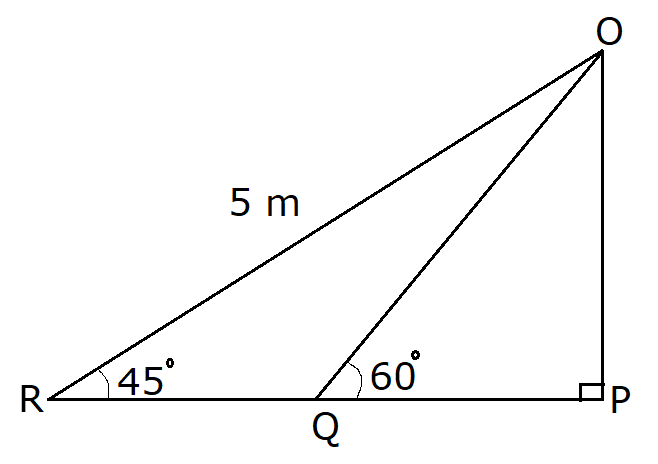In the diagram, $|OR| = 5m$, $\angle ORP = 45^\circ$, $\angle OQP = 60^\circ$ and $\angle OPR = 90^\circ$.
Find the distance $QP$, leaving your answer in surd form.

Leaving the answer in surd form implies we do not have to use a calculator.
We shall use the trigonometric functions of those special angles in surd form.
Absolutely no decimals

$\underline{\triangle ORP} \\[3ex] \cos 45^\circ = \dfrac{|RP|}{5} ... SOHCAHTOA \\[5ex] |RP| = 5 * \cos 45 \\[3ex] \cos 45 = \dfrac{\sqrt{2}}{2} ... unit\:\: circle\:\: trig \\[5ex] |RP| = 5 * \dfrac{\sqrt{2}}{2} \\[5ex] |RP| = \dfrac{5\sqrt{2}}{2} \\[5ex] |OR|^2 = |RP|^2 + |OP|^2 ... Pythagorean\:\: Theorem \\[3ex] |OP|^2 = |OR|^2 - |RP|^2 \\[3ex] |OR| = 5 \\[3ex] |OP|^2 = 5^2 - \left(\dfrac{5\sqrt{2}}{2}\right)^2 \\[5ex] \left(\dfrac{5\sqrt{2}}{2}\right)^2 = \dfrac{5^2 * (\sqrt{2})^2}{2^2} = \dfrac{25 * 2}{4} = \dfrac{25}{2} \\[5ex] |OP|^2 = 25 - \dfrac{25}{2} \\[5ex] |OP|^2 = \dfrac{50}{2} - \dfrac{25}{2} = \dfrac{50 - 25}{2} = \dfrac{25}{2} \\[5ex] |OP| = \sqrt{\dfrac{25}{2}} \\[5ex] |OP| = \dfrac{\sqrt{25}}{\sqrt{2}} = \dfrac{5}{\sqrt{2}} \\[5ex] \underline{OQP} \\[3ex] \tan 60 = \dfrac{|OP|}{|QP|} \\[5ex] |QP| * \tan 60 = |OP| \\[3ex] |QP| = \dfrac{|OP|}{\tan 60} \\[5ex] |QP| = |OP| \div \tan 60 \\[3ex] \tan 60 = \sqrt{3} ... unit\:\: circle\:\: trig \\[3ex] |QP| = \dfrac{5}{\sqrt{2}} \div \sqrt{3} \\[5ex] |QP| = \dfrac{5}{\sqrt{2}} * \dfrac{1}{\sqrt{3}} \\[5ex] |QP| = \dfrac{5 * 1}{\sqrt{2} * \sqrt{3}} \\[5ex] |QP| = \dfrac{5}{\sqrt{2 * 3}} \\[5ex] |QP| = \dfrac{5}{\sqrt{6}} \\[5ex] |QP| = \dfrac{5}{\sqrt{6}} * \dfrac{\sqrt{6}}{\sqrt{6}} \\[5ex] |QP| = \dfrac{5 * \sqrt{6}}{\sqrt{6} * \sqrt{6}} \\[5ex] |QP| = \dfrac{5\sqrt{6}}{6}$
(12.) ACT In right triangle $\triangle ABC$ shown below, $\sin C = \dfrac{5}{7}$ and the length of $\overline{AB}$ is 14 inches.
What is the length, in inches of $\overline{AC}$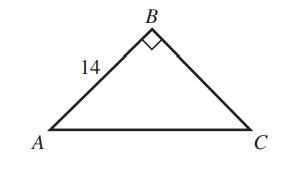$F.\;\; \sqrt{24} \\[3ex] G.\;\; \sqrt{74} \\[3ex] H.\;\; 10 \\[3ex] J.\;\; 19.6 \\[3ex] K.\;\; 24 \\[3ex]$

$SOH:CAH:TOA \\[3ex] \sin C = \dfrac{opp}{hyp} = \dfrac{5}{7} = \dfrac{\overline{AB}}{\overline{AC}} \\[5ex] \dfrac{5}{7} = \dfrac{14}{\overline{AC}} \\[5ex] Cross\;\;multiply \\[3ex] 5 * \overline{AC} = 7(14) \\[3ex] 5 * \overline{AC} = 98 \\[3ex] \overline{AC} = \dfrac{98}{5} \\[5ex] \overline{AC} = 19.6\;inches$
(13.) Use the angle in the unit circle to find the exact values of these trigonometric functions.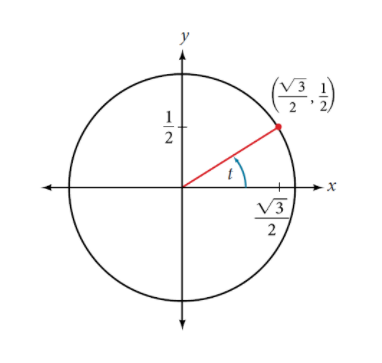$(a.)\;\; \sin t \\[3ex] (b.)\;\; \cos t \\[3ex] (c.)\;\; \tan t \\[3ex] (d.)\;\; \csc t \\[3ex] (e.)\;\; \sec t \\[3ex] (f.)\;\; \cot t \\[3ex]$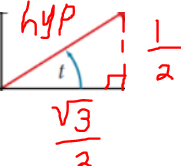$hyp^2 = \left(\dfrac{1}{2}\right)^2 + \left(\dfrac{\sqrt{3}}{2}\right)^2 ... Pythagorean\:\: Theorem \\[5ex] hyp^2 = \dfrac{1}{4} + \dfrac{3}{4} \\[5ex] hyp^2 = \dfrac{4}{4} \\[5ex] hyp^2 = 1 \\[3ex] hyp = \pm \sqrt{1} \\[3ex] hypotenuse\;\;is\;\;not\;\;negative \\[3ex] hyp = 1 \\[3ex] \underline{Based\;\;on\;\;that\;\;triangle} \\[3ex] opp = \dfrac{1}{2} \\[5ex] adj = \dfrac{\sqrt{3}}{2} \\[5ex] hyp = 1 \\[3ex] \underline{SOH:CAH:TOA} \\[3ex] (a.) \\[3ex] \sin t \\[3ex] = \dfrac{opp}{hyp} \\[5ex] = \dfrac{1}{2} \div 1 \\[5ex] = \dfrac{1}{2} \\[5ex] (b.) \\[3ex] \cos t \\[3ex] = \dfrac{adj}{hyp} \\[5ex] = \dfrac{\sqrt{3}}{2} \div 1 \\[5ex] = \dfrac{\sqrt{3}}{2} \\[5ex] (c.) \\[3ex] \tan t \\[3ex] = \dfrac{opp}{adj} \\[5ex] = \dfrac{1}{2} \div \dfrac{\sqrt{3}}{2} \\[5ex] = \dfrac{1}{2} * \dfrac{2}{\sqrt{3}} \\[5ex] = \dfrac{1}{\sqrt{3}} \\[5ex] = \dfrac{1}{\sqrt{3}} * \dfrac{\sqrt{3}}{\sqrt{3}} \\[5ex] = \dfrac{\sqrt{3}}{3} \\[5ex] \underline{COSECHO:SECHA:COTANAO} \\[3ex] (d.) \\[3ex] \csc t \\[3ex] = \dfrac{hyp}{opp} \\[5ex] = 1 \div \dfrac{1}{2} \\[5ex] = 1 * \dfrac{2}{1} \\[5ex] = 2 \\[3ex] (e.) \\[3ex] \sec t \\[3ex] = \dfrac{hyp}{adj} \\[5ex] = 1 \div \dfrac{\sqrt{3}}{2} \\[5ex] = 1 * \dfrac{2}{\sqrt{3}} \\[5ex] = \dfrac{2}{\sqrt{3}} * \dfrac{\sqrt{3}}{\sqrt{3}} \\[5ex] = \dfrac{2\sqrt{3}}{3} \\[5ex] (f.) \\[3ex] \cot t \\[3ex] = \dfrac{adj}{opp} \\[5ex] = \dfrac{\sqrt{3}}{2} \div \dfrac{1}{2} \\[5ex] = \dfrac{\sqrt{3}}{2} * \dfrac{2}{1} \\[5ex] = \sqrt{3}$
(14.) JAMB In a right angled triangle, if $\tan\theta = \dfrac{3}{4}$, what is $\cos\theta - \sin\theta$?

$A.\;\; \dfrac{3}{5} \\[5ex] B.\;\; \dfrac{2}{5} \\[5ex] C.\;\; \dfrac{1}{5} \\[5ex] D.\;\; \dfrac{4}{5} \\[5ex]$

$\tan\theta = \dfrac{3}{4} = \dfrac{opp}{adj} ... SOHCAHTOA \\[5ex] opp = 3 \\[3ex] adj = 4 \\[3ex] hyp^2 = opp^2 + adj^2 ... Pythagorean\:\: Theorem \\[3ex] hyp^2 = 3^2 + 4^2 \\[3ex] hyp^2 = 9 + 16 = 25 \\[3ex] hyp = \sqrt{25} \\[3ex] hyp = 5 \\[3ex] \cos\theta = \dfrac{adj}{hyp} = \dfrac{4}{5} ... SOHCAHTOA \\[5ex] \sin\theta = \dfrac{opp}{hyp} = \dfrac{3}{5} ... SOHCAHTOA \\[5ex] \cos\theta - \sin\theta = \dfrac{4}{5} - \dfrac{3}{5} = \dfrac{4 - 3}{5} \\[5ex] \cos\theta - \sin\theta = \dfrac{1}{5}$
(15.)

(16.) ACT What is the length, in inches, of the hypotenuse of a right triangle with a leg that is 9 inches long and a leg that is 2 inches long?

$A.\;\; \sqrt{22} \\[3ex] B.\;\; \sqrt{77} \\[3ex] C.\;\; \sqrt{85} \\[3ex] D.\;\; 5.5 \\[3ex] E.\;\; 11 \\[3ex]$

$hyp^2 = leg^2 + leg^2 ...Pythagorean\:\: Theorem \\[3ex] leg = 9 \\[3ex] leg = 2 \\[3ex] hyp^2 = 9^2 + 2^2 \\[3ex] hyp^2 = 81 + 4 = 85 \\[3ex] hyp = \sqrt{85}$
(17.)

(18.) curriculum.gov.mt The hypotenuse of a right-angled triangle is 25 cm long.
One of the adjacent sides is 15 cm long.
How long is the third side?

$hyp^2 = leg^2 + leg^2 ...Pythagorean\:\: Theorem \\[3ex] 25^2 = leg^2 + 15^2 \\[3ex] leg^2 + 15^2 = 25^2 \\[3ex] leg^2 + 225 = 625 \\[3ex] leg^2 = 625 - 225 \\[3ex] leg^2 = 400 \\[3ex] leg = \sqrt{400} \\[3ex] leg = 20\;cm$
(19.) NZQA Spiders often use trees to support their webs.
Angle JKM = 32°   KJ = 12.5 cm   JH = 21.2 cm   NH = 9.4 cm
Angle KMJ = Angle JNH = 90°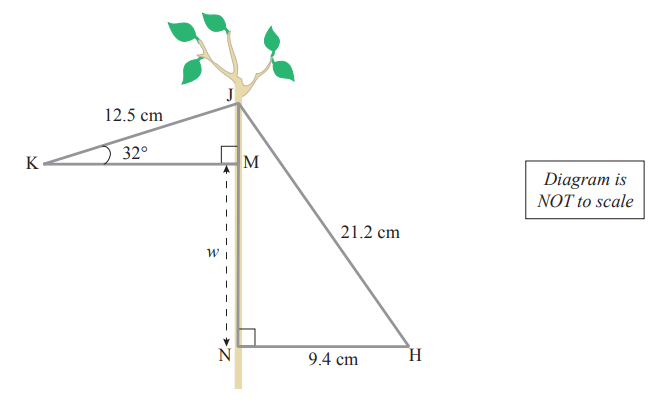Calculate the length, w, from M to N

$\underline{\triangle KJM} \\[3ex] \sin 32 = \dfrac{|JM|}{12.5} ...SOHCAHTOA \\[5ex] 12.5 \sin 32 = |JM| \\[3ex] |JM| = 12.5 \sin 32 \\[3ex] |JM| = 12.5(0.5299192642) \\[3ex] |JM| = 6.623990803\;cm \\[3ex] \underline{\triangle JNH} \\[3ex] 21.2^2 = |JN|^2 + 9.4^2 ...Pythagorean\;\;theorem \\[3ex] |JN|^2 + 9.4^2 = 21.2^2 \\[3ex] |JN|^2 = 21.2^2 - 9.4^2 \\[3ex] |JN|^2 = 449.44 - 88.36 \\[3ex] |JN|^2 = 361.08 \\[3ex] |JN| = \sqrt{361.08} \\[3ex] |JN| = 19.00210515\;cm \\[3ex] But: \\[3ex] |JN| = |JM| + |MN|...diagram \\[3ex] 19.00210515 = 6.623990803 + w \\[3ex] 6.623990803 + w = 19.00210515 \\[3ex] w = 19.00210515 - 6.623990803 \\[3ex] w = 12.37811434 \\[3ex] w \approx 12.4\;cm$
(20.) ACT In right triangle $\triangle ABC$ shown below, $\sin C = \dfrac{2}{3}$ and the length of $\overline{AB}$ is 6 inches.
What is the length, in inches of $\overline{AC}$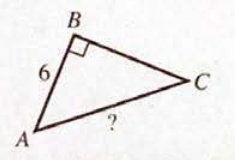$A.\;\; \sqrt{5} \\[3ex] B.\;\; \sqrt{13} \\[3ex] C.\;\; 4 \\[3ex] D.\;\; 5 \\[3ex] E.\;\; 9 \\[3ex]$

$SOH:CAH:TOA \\[3ex] \sin C = \dfrac{opp}{hyp} = \dfrac{2}{3} = \dfrac{\overline{AB}}{\overline{AC}} \\[5ex] \dfrac{2}{3} = \dfrac{6}{\overline{AC}} \\[5ex] Cross\;\;multiply \\[3ex] 2 * \overline{AC} = 3(6) \\[3ex] \overline{AC} = \dfrac{3(6)}{2} \\[5ex] \overline{AC} = 3(3) \\[3ex] \overline{AC} = 9\;inches$

(21.)

(22.) ACT In the right triangle shown below, the length of $\overline{AC}$ is 4 mm and the length of $\overline{BC}$ is 3 mm.
For $\angle A$, the value of which of the following trigonometric expressions is $\dfrac{3}{5}$?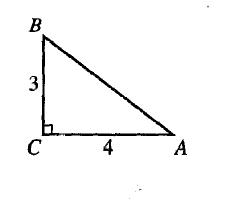$A.\;\; \sin A \\[3ex] B.\;\; \cos A \\[3ex] C.\;\; \tan A \\[3ex] D.\;\; \csc A \\[3ex] E.\;\; \cot A \\[3ex]$

$hyp^2 = leg^2 + leg^2 ...Pythagorean\:\: Theorem \\[3ex] \overline{AB}^2 = \overline{AC}^2 + \overline{BC}^2 \\[3ex] \overline{AB}^2 = 4^2 + 3^2 \\[3ex] \overline{AB}^2 = 16 + 9 \\[3ex] \overline{AB}^2 = 25 \\[3ex] \overline{AB} = \sqrt{25} \\[3ex] \overline{AB} = 5 \\[3ex] SOHCAHTOA \\[3ex] For\;\; \angle A: \\[3ex] opp = 3 \\[3ex] hyp = 5 \\[3ex] \dfrac{3}{5} = \sin A$
(23.) NZQA The section of one spider web shown below has two connecting right-angled triangles.
Angle PQS = 63°                  QS = 16.4 cm
Angle RQS = Angle QPS = 90°       QR = 7.5 cm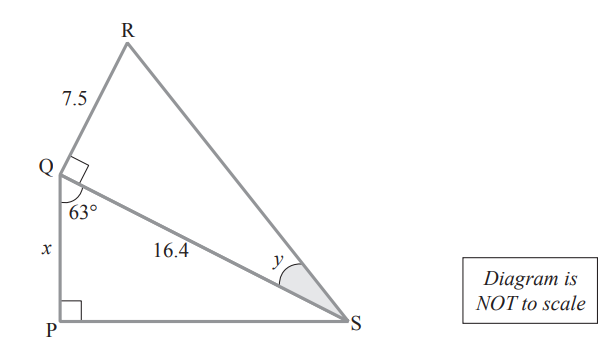(i) Calculate the length, x, from P to Q

(ii) Calculate the size, y, of angle QSR

$(i) \\[3ex] \underline{\triangle PQS} \\[3ex] 63 + 90 + \angle QSP = 180^\circ ...sum\;\;of\;\;\angle s\;\;of\;\;\triangle PQS \\[3ex] 153 + \angle QSP = 180 \\[3ex] \angle QSP = 180 - 153 \\[3ex] \angle QSP = 27^\circ \\[3ex] \dfrac{x}{\sin \angle QSP} = \dfrac{16.4}{\sin 90} ...Sine\;\;Law \\[5ex] \dfrac{x}{\sin 27} = \dfrac{16.4}{1} \\[5ex] x = 16.4 \sin 27 \\[3ex] x = 16.4(0.4539904997) \\[3ex] x = 7.445444196 \\[3ex] x \approx 7.45\;cm \\[3ex] (ii) \\[3ex] \underline{\triangle RQS} \\[3ex] |RS|^2 = 7.5^2 + 16.4^2...Pythagorean\;\;theorem \\[3ex] |RS|^2 = 56.25 + 268.96 \\[3ex] |RS|^2 = 325.21 \\[3ex] |RS| = \sqrt{325.21} \\[3ex] |RS| = 18.03357979 \\[3ex] \dfrac{\sin y}{7.5} = \dfrac{\sin 90}{|RS|} ... Sine\;\;Law \\[5ex] \dfrac{\sin y}{7.5} = \dfrac{1}{18.03357979} \\[5ex] \sin y = \dfrac{7.5 * 1}{18.03357979} \\[5ex] \sin y = \dfrac{7.5}{18.03357979} \\[5ex] \sin y = 0.4158908041 \\[3ex] y = \sin^{-1}(0.4158908041) \\[3ex] y = 24.5754272 \\[3ex] y \approx 25^\circ$
(24.) ACT Royce plans to construct a triangular flower bed on the corner of his property where a sidewalk forms a right angle.
The flower bed and the lengths, in feet, of 2 of its sides are shown in the figure below.
The flower bed will be enclosed by a garden fence that is set up along its entire perimeter.
To the nearest foot, how many feet of garden fence will enclose the flower bed?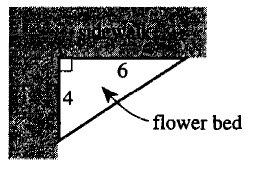$F.\;\; 12 \\[3ex] G.\;\; 14 \\[3ex] H.\;\; 16 \\[3ex] J.\;\; 17 \\[3ex] K.\;\; 20 \\[3ex]$

$hyp^2 = leg^2 + leg^2 ...Pythagorean\:\: Theorem \\[3ex] hyp^2 = 4^2 + 6^2 \\[3ex] hyp^2 = 16 +36 \\[3ex] hyp^2 = 52 \\[3ex] hyp = \sqrt{52} = 7.211102551 \\[3ex] To\;\;enclose\;\;the\;\;flower\;\;bed \implies Perimeter \\[3ex] Perimeter = 4 + 6 + 7.211102551 \\[3ex] Perimeter = 17.21110255 \\[3ex] To\;\;the\;\;nearest\;\;foot: \\[3ex] Perimeter \approx 17\;\;feet$
(25.)

(26.) ACT In $\triangle XYZ$, the measure of $\angle X$ is $90^\circ$, the measure of $\angle Z$ is $\theta$, $XY = 12$ units and $\tan \theta = \dfrac{4}{9}$
What is the area of $\triangle XYZ$, in square units?

$F.\;\; 162 \\[3ex] G.\;\; 324 \\[3ex] H.\;\; 2\sqrt{65} \\[3ex] J.\;\; 6\sqrt{585} \\[3ex] K.\;\; 12\sqrt{585} \\[3ex]$

Let us draw the triangle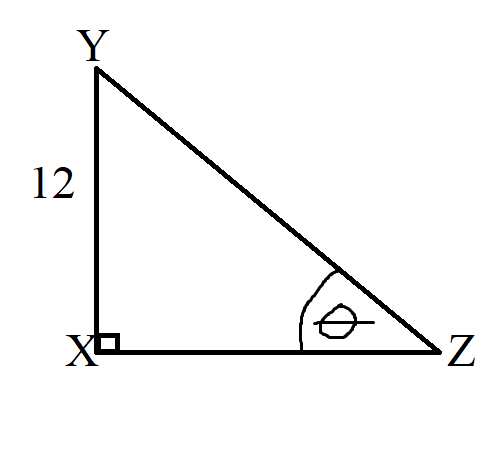$\theta^\circ \\[3ex] \tan \theta = \dfrac{opp}{adj} = \dfrac{4}{9} = \dfrac{12}{XZ}...SOHCAHTOA \\[5ex] \dfrac{4}{9} = \dfrac{12}{XZ} \\[5ex] Cross\;\;Multiply \\[3ex] 4 * XZ = 9 * 12 \\[3ex] XZ = \dfrac{9 * 12}{4} \\[5ex] XZ = 9(3) = 27 \\[3ex] Area\;\;of\;\;\triangle XYZ \\[3ex] = \dfrac{1}{2} * XY * XZ * \sin X \\[5ex] = \dfrac{1}{2} * 12 * 27 * \sin 90^\circ \\[5ex] = 6 * 27 * 1 \\[3ex] = 162\;\;square\;\;unit$
(27.) Use the angle in the unit circle to find the exact values of these trigonometric functions.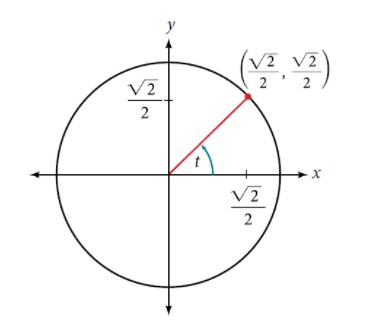$(a.)\;\; \sin t \\[3ex] (b.)\;\; \cos t \\[3ex] (c.)\;\; \tan t \\[3ex] (d.)\;\; \csc t \\[3ex] (e.)\;\; \sec t \\[3ex] (f.)\;\; \cot t \\[3ex]$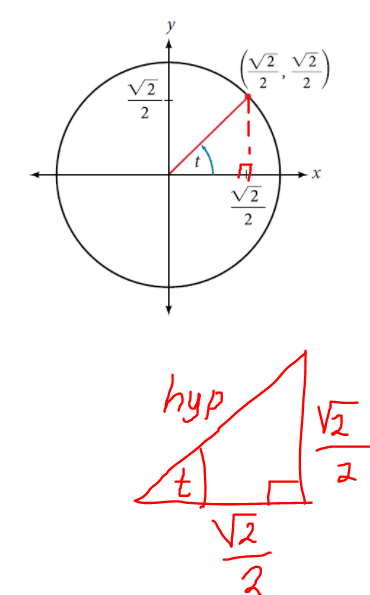$hyp^2 = \left(\dfrac{\sqrt{2}}{2}\right)^2 + \left(\dfrac{\sqrt{2}}{2}\right)^2 ... Pythagorean\:\: Theorem \\[5ex] hyp^2 = \dfrac{2}{4} + \dfrac{2}{4} \\[5ex] hyp^2 = \dfrac{4}{4} \\[5ex] hyp^2 = 1 \\[3ex] hyp = \pm \sqrt{1} \\[3ex] hypotenuse\;\;is\;\;not\;\;negative \\[3ex] hyp = 1 \\[3ex] \underline{Based\;\;on\;\;that\;\;triangle} \\[3ex] opp = \dfrac{\sqrt{2}}{2} \\[5ex] adj = \dfrac{\sqrt{2}}{2} \\[5ex] hyp = 1 \\[3ex] \underline{SOH:CAH:TOA} \\[3ex] (a.) \\[3ex] \sin t \\[3ex] = \dfrac{opp}{hyp} \\[5ex] = \dfrac{\sqrt{2}}{2} \div 1 \\[5ex] = \dfrac{\sqrt{2}}{2} \\[5ex] (b.) \\[3ex] \cos t \\[3ex] = \dfrac{adj}{hyp} \\[5ex] = \dfrac{\sqrt{2}}{2} \div 1 \\[5ex] = \dfrac{\sqrt{2}}{2} \\[5ex] (c.) \\[3ex] \tan t \\[3ex] = \dfrac{opp}{adj} \\[5ex] = \dfrac{\sqrt{2}}{2} \div \dfrac{\sqrt{2}}{2} \\[5ex] = 1 \\[3ex] \underline{COSECHO:SECHA:COTANAO} \\[3ex] (d.) \\[3ex] \csc t \\[3ex] = \dfrac{hyp}{opp} \\[5ex] = 1 \div \dfrac{\sqrt{2}}{2} \\[5ex] = 1 * \dfrac{2}{\sqrt{2}} \\[5ex] = \dfrac{2}{\sqrt{2}} \\[5ex] = \dfrac{2}{\sqrt{2}} * \dfrac{\sqrt{2}}{\sqrt{2}} \\[5ex] = \dfrac{2\sqrt{2}}{2} \\[5ex] = \sqrt{2} \\[3ex] (e.) \\[3ex] \sec t \\[3ex] = \dfrac{hyp}{adj} \\[5ex] = 1 \div \dfrac{\sqrt{2}}{2} \\[5ex] = \sqrt{2} \\[3ex] (f.) \\[3ex] \cot t \\[3ex] = \dfrac{adj}{opp} \\[5ex] = \dfrac{\sqrt{2}}{2} \div \dfrac{\sqrt{2}}{2} \\[5ex] = 1$
(28.) ACT In the figure shown below, a ladder 15 feet long forms an angle of $56^\circ$ with the level ground as it leans against the vertical side of a building.
The distance along the building, in feet, between the ground and the top of the ladder is equal to which of the following expressions?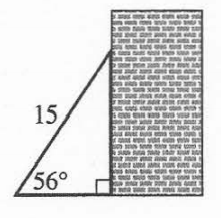$F.\;\; \dfrac{15}{2} \\[5ex] G.\;\; \dfrac{15\sqrt{3}}{2} \\[5ex] H.\;\; 15 \sin 56^\circ \\[3ex] J.\;\; 15 \cos 56^\circ \\[3ex] K.\;\; 15 \tan 56^\circ \\[3ex]$

$Let\;\;the\;\;distance = opp \\[3ex] SOH:CAH:TOA \\[3ex] \sin 56 = \dfrac{opp}{15} \\[5ex] opp = 15\sin 56^\circ$
(29.)

(30.) ACT What is the area, in square meters, of a right triangle with sides of length 8 meters, 15 meters, and 17 meters?

$F.\;\; 40 \\[3ex] G.\;\; 60 \\[3ex] H.\;\; 68 \\[3ex] J.\;\; 120 \\[3ex] K.\;\; 127\dfrac{1}{2} \\[5ex]$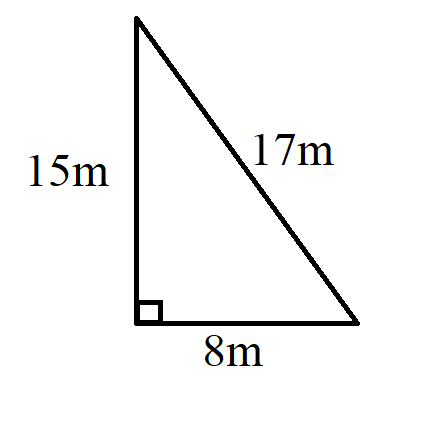$hyp\;\;faces\;\;the\;\;right\;\;\angle \\[3ex] hyp = longest\;\;side = 17\;meters \\[3ex] Sides\;\;that\;\;include\;\;90^\circ = 8\;meters\;\;and\;\;15\;meters \\[3ex] Area = \dfrac{1}{2} * 8 * 15 * \sin 90 \\[5ex] = 4 * 15 * 1 \\[3ex] = 60\;meters^2$
(31.) NZQA Angle NPM = 42°.
Angle NQM = 36°.
Angle MNP = 90°.
MN = c metres.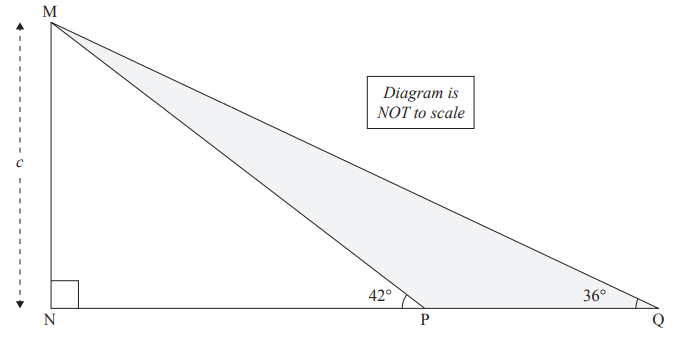Show all working to 4 decimal places.

$\underline{\triangle MNP} \\[3ex] \sin \angle NPM = \dfrac{|MN|}{|MP|}...SOHCAHTOA \\[5ex] \sin 42^\circ = \dfrac{c}{|MP|} \\[5ex] |MP| = \dfrac{c}{\sin 42} \\[5ex] |MP| \approx 1.4945c \\[3ex] \tan \angle NPM = \dfrac{|MN|}{|NP|}...SOHCAHTOA \\[5ex] \tan 42^\circ = \dfrac{c}{|NP|} \\[5ex] |NP| = \dfrac{c}{\tan 42} \\[5ex] \underline{\triangle MNQ} \\[3ex] \sin \angle NQM = \dfrac{|MN|}{|MQ|}...SOHCAHTOA \\[5ex] \sin 36^\circ = \dfrac{c}{|MQ|} \\[5ex] |MQ| = \dfrac{c}{\sin 36} \\[5ex] |MQ| \approx 1.7013c \\[3ex] \tan \angle NQM = \dfrac{|MN|}{|NQ|}...SOHCAHTOA \\[5ex] \tan 36^\circ = \dfrac{c}{|NQ|} \\[5ex] |NQ| = \dfrac{c}{\tan 36} \\[5ex] |PQ| = |NQ| - |NP| ...diagram \\[3ex] |PQ| = \dfrac{c}{\tan 36} - \dfrac{c}{\tan 42} \\[5ex] |PQ| = \dfrac{c\tan 42 - c\tan 36}{\tan 36\tan 42} \\[5ex] |PQ| = \dfrac{c(\tan 42 - \tan 36)}{\tan 36\tan 42} \\[5ex] |PQ| = \dfrac{c(0.9004040 - 0.7265425)}{0.7265425(0.9004040)} \\[5ex] |PQ| = \dfrac{c(0.1738615)}{0.6541818} \\[5ex] |PQ| \approx 0.2658c \\[3ex] Perimeter\;\;of\;\;shaded\;\;region\;\;MPQ \\[3ex] = |MP| + |MQ| + |PQ| \\[3ex] \approx 1.4945c + 1.7013c + 0.2658c \\[3ex] \approx 3.4616c$
(32.) CSEC In the diagram below, not drawn to scale, EFGH is a rectangle.
The point D on HG is such that $ED = DG = 12\;cm$ and $G\hat{D}F = 43^\circ$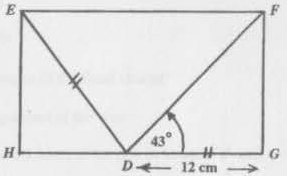Calculate correct to one decimal place
(i.) the length of GF
(ii.) the length of HD
(iii.) the size of the angle HDE

$(i.) \\[3ex] \underline{\triangle DFG} \\[3ex] SOH:CAH:TOA \\[3ex] \tan 43 = \dfrac{|GF|}{12} \\[5ex] 12\tan 43 = |GF| \\[3ex] |GF| = 12 * \tan 43 \\[3ex] |GF| = 12(0.9325150861) \\[3ex] |GF| = 11.19018103 \\[3ex] |GF| \approx 11.2\;cm \\[3ex] (ii.) \\[3ex] \underline{\triangle HDE} \\[3ex] hyp^2 = leg^2 + leg^2 ...Pythagorean\;\;Theorem \\[3ex] hyp = |ED| = 12\;cm \\[3ex] HE = GF ...opposite\;\;sides\;\;of\;\;a\;\;rectangle\;\;are\;\;equal \\[3ex] \implies leg = HE = 11.19018103 \\[3ex] |ED|^2 = |HE|^2 + |HD|^2 \\[3ex] 12^2 = (11.19018103)^2 + |HD|^2 \\[3ex] |HD|^2 + 11.19018103^2 = 12^2 \\[3ex] |HD|^2 = 12^2 - 11.19018103^2 \\[3ex] |HD|^2 = 144 - 125.2201515 \\[3ex] |HD|^2 = 18.7798485 \\[3ex] |HD| = \sqrt{18.7798485} \\[3ex] |HD| = 4.333572256 \\[3ex] |HD| \approx 4.3\;cm \\[3ex] (iii.) \\[3ex] \underline{\triangle HDE} \\[3ex] SOH:CAH:TOA \\[3ex] \cos H\hat{D}E = \dfrac{|HD|}{|ED|} \\[5ex] \cos H\hat{D}E = \dfrac{4.333572256}{12} \\[5ex] \cos H\hat{D}E = 0.3611310214 \\[3ex] H\hat{D}E = \cos^{-1}(0.3611310214) \\[3ex] H\hat{D}E = 68.83032783 \\[3ex] H\hat{D}E \approx 68.8^\circ$
(33.)

(34.) ACT In the right triangle below, how long is side $\overline{AB}$ ?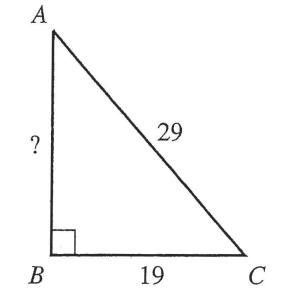$F.\;\; \sqrt{29^2 - 19^2} \\[3ex] G.\;\; \sqrt{29^2 + 19^2} \\[3ex] H.\;\; 29^2 - 19^2 \\[3ex] J.\;\; 29^2 + 19^2 \\[3ex] K.\;\; 29 - 19 \\[3ex]$

$\overline{AB}^2 + \overline{BC}^2 = \overline{AC}^2 ...Pythagorean\;\;Theorem \\[3ex] \overline{AB}^2 + 19^2 = 29^2 \\[3ex] \overline{AB}^2 = 29^2 - 19^2$
(35.)

(36.) CSEC The diagram below shows the triangle PQR in which angle QPR = 62°, angle PQR = 90° and PR = 11 cm.Calculate

(i) the size of angle PRQ

(ii) the length of the side RQ

$(i) \\[3ex] \angle PRQ + \angle RPQ + \angle PQR = 180^\circ ...sum\;\;of\;\;\angle s\;\;of\;\;\triangle RPQ \\[3ex] \angle PRQ + 62 + 90 = 180 \\[3ex] \angle PRQ + 152 = 180 \\[3ex] \angle PRQ = 180 - 152 \\[3ex] \angle PRQ = 28^\circ \\[3ex] (ii) \\[3ex] \sin 62 = \dfrac{|RQ|}{11} ...SOHCAHTOA \\[5ex] 11\sin 62 = |RQ| \\[3ex] |RQ| = 11 * \sin 62 \\[3ex] |RQ| = 11(0.8829475929) \\[3ex] |RQ| = 9.712423521\;cm$
(37.)

(38.) SAT-NC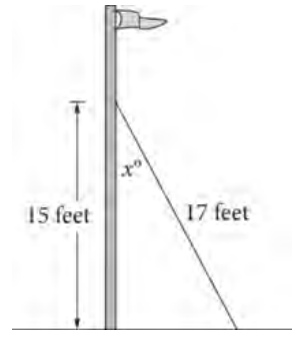The figure shows the mast of a boat that is installed perpendicular to the deck of the boat.
The mast is secured by a rope that is anchored to the deck.
The rope measures 17 feet long and makes an angle of x° with the mast.
The point where the rope is attached to the mast is 15 feet above the deck.
What is the value of tan(x°)?

perpendicular tp the deck of the boat means that is at a right angle to the deck of the boat.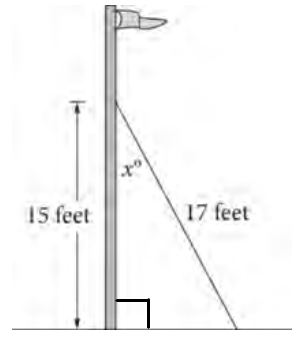$leg^2 + leg^2 = hyp^2 ...Pythagorean\;\;Theorem \\[3ex] leg^s + 15^2 = 17^2 \\[3ex] leg^2 + 225 = 289 \\[3ex] leg^2 = 289 - 225 \\[3ex] leg^2 = 64 \\[3ex] leg = \sqrt{64} \\[3ex] leg = 8\;feet \\[3ex] \tan x^\circ = \dfrac{8}{15} ...SOHCAHTOA$
(39.) NZQA Spider web strands link together to increase their strength.
Angle ZVY = 31°        Angle VYZ = 90°
Angle ZWY = 41°       VW = 36 cm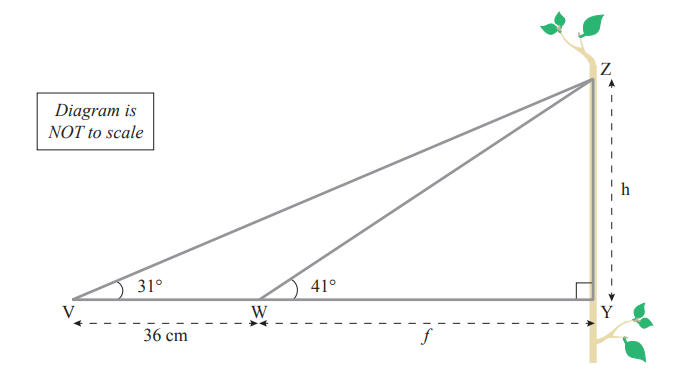Calculate the length, f, from W to Y.

$\underline{\triangle ZWY} \\[3ex] \tan 41 = \dfrac{h}{f} ...SOHCAHTOA \\[5ex] f\tan 41 = h ...eqn.(1) \\[3ex] \underline{\triangle ZVY} \\[3ex] \tan 31 = \dfrac{h}{36 + f} ... SOHCAHTOA \\[5ex] (36 + f)\tan 31 = h ... eqn.(2) \\[3ex] h = h \implies eqn.(1) = eqn.(2) \\[3ex] f\tan 41 = (36 + f)\tan 31 \\[3ex] f\tan 41 = \tan 31(36 + f) \\[3ex] f\tan 41 = 36\tan 31 + f\tan 31 \\[3ex] f\tan 41 - f\tan 31 = 36\tan 31 \\[3ex] f(\tan 41 - \tan 31) = 36\tan 31 \\[3ex] f = \dfrac{36\tan 31}{\tan 41 - \tan 31} \\[5ex] f = \dfrac{36(0.600860619)}{0.8692867378 - 0.600860619} \\[5ex] f = \dfrac{21.63098228}{0.2684261188} \\[5ex] f = 80.58449147 \\[3ex] f \approx 81\;cm$
(40.) ACT From a hot air balloon, the angle between a radio antenna straight below and the base of the library downtown is 57°, as shown below.
If the distance between the radio antenna and the library is 1.3 miles, how many miles high is the balloon?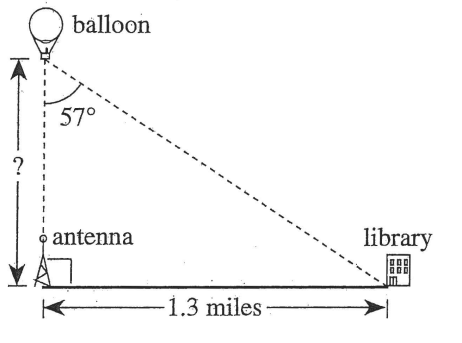$A.\;\; 1.3\sin 57^\circ \\[3ex] B.\;\; 1.3\tan 57^\circ \\[3ex] C.\;\; \dfrac{1.3}{\sin 57^\circ} \\[5ex] D.\;\; \dfrac{1.3}{\cos 57^\circ} \\[5ex] E.\;\; \dfrac{1.3}{\tan 57^\circ} \\[5ex]$

$\tan 57^\circ = \dfrac{1.3}{adj}...SOHCAHTOA \\[5ex] adj * \tan 57^\circ = 1.3 \\[3ex] adj = \dfrac{1.3}{\tan 57^\circ}$

(41.) SAT-C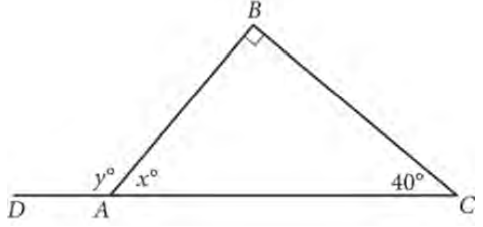In triangle ABC, $\overline{CA}$ is extended to point D.
What is the value of y?

$A)\;\; 50 \\[3ex] B)\;\; 115 \\[3ex] C)\;\; 130 \\[3ex] D)\;\; 140 \\[3ex]$

$y = 90 + 40 ...exterior\;\;\angle\;\;of\;\;a\;\;\triangle = sum\;\;of\;\;the\;\;two\;\;interior\;\;opposite\;\;\angle s \\[3ex] y = 130^\circ$
(42.) ACT In the right triangle below, if $\overline{BC}$ is 4 inches long, how many inches long is $\overline{AB}$?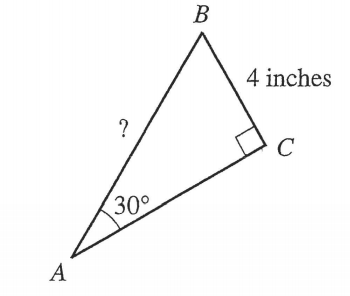$A.\;\; 4 \\[3ex] B.\;\; 4\sqrt{2} \\[3ex] C.\;\; 4\sqrt{3} \\[3ex] D.\;\; 6 \\[3ex] E.\;\; 8 \\[3ex]$

$\overline{AB} = hyp \\[3ex] \sin 30 = \dfrac{1}{2} ...Special\;\;\angle \\[3ex] \sin 30 = \dfrac{4}{hyp}...SOHCAHTOA \\[5ex] hyp * \sin 30 = 4 \\[3ex] hyp = \dfrac{4}{\sin 30} \\[5ex] hyp = 4 \div \sin 30 \\[3ex] hyp = 4 \div \dfrac{1}{2} \\[5ex] hyp = 4 * \dfrac{2}{1} \\[5ex] hyp = 8 \\[3ex] \therefore \overline{AB} = 8\;inches$
(43.)

(44.)

(45.)

(46.) ACT The sides of a triangle are 5, 12, and 13 inches long.
What is the angle between the 2 shortest sides?

$A.\;\; 30^\circ \\[3ex] B.\;\; 45^\circ \\[3ex] C.\;\; 60^\circ \\[3ex] D.\;\; 90^\circ \\[3ex] E.\;\; 120^\circ \\[3ex]$

$Because: \\[3ex] 13^2 = 5^2 + 12^2 \\[3ex] 169 = 25 + 144 \\[3ex] hyp^2 = leg^2 + leg^2 \\[3ex] The\;\;\triangle\;\;is\;\;a\;\;right\;\;\triangle \\[3ex] Because: \\[3ex] the\;\;hypotenuse\;\;is\;\;the\;\;side\;\;facing\;\;90^\circ \\[3ex] this\;\;means\;\;that\;\;90^\circ\;\;is\;\;included\;\;between\;\;the\;\;other\;\;2\;\;sides \\[3ex] \therefore \angle = 90^\circ \\[3ex]$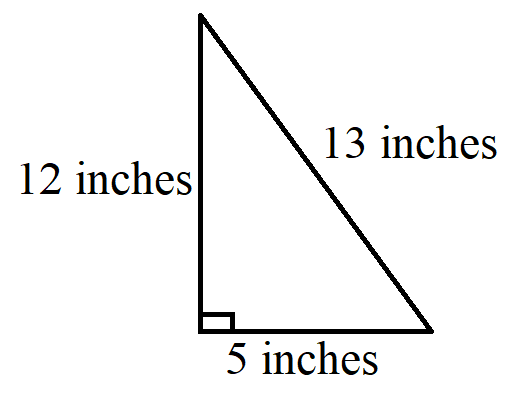(47.)

(48.)

(49.)

(50.) ACT Right triangle $\triangle ABC$ and its side lengths given in inches are shown below.
What is $\sin B$?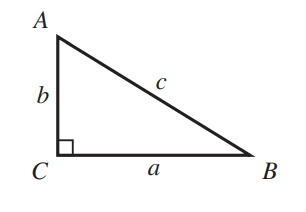$A.\;\; \dfrac{a}{b} \\[5ex] B.\;\; \dfrac{a}{c} \\[5ex] C.\;\; \dfrac{b}{c} \\[5ex] D.\;\; \dfrac{b}{c} \\[5ex] E.\;\; \dfrac{c}{a} \\[5ex]$

$\sin B \\[3ex] = \dfrac{opp}{hyp} ...SOHCAHCAH \\[5ex] = \dfrac{b}{c}$
(51.)

(52.)

(53.)

(54.)

(55.)

(56.) ACT In right triangle $\triangle ABC$ shown below, the given lengths are in millimeters.
What is $\sin A$?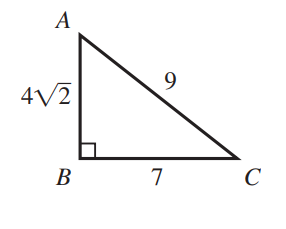$A.\;\; \dfrac{4\sqrt{2}}{9} \\[5ex] B.\;\; \dfrac{4\sqrt{2}}{7} \\[5ex] C.\;\; \dfrac{7\sqrt{2}}{8} \\[5ex] D.\;\; \dfrac{7}{9} \\[5ex] E.\;\; \dfrac{9}{7} \\[5ex]$

$\sin A = \dfrac{opp}{hyp} ... SOHCAHTOA \\[5ex] \sin A = \dfrac{7}{9}$
(57.)

(58.)

(59.)

(60.)

(61.)

(62.)